Ex 10.1

Chapter 10 Class 12 Vector Algebra
Serial order wise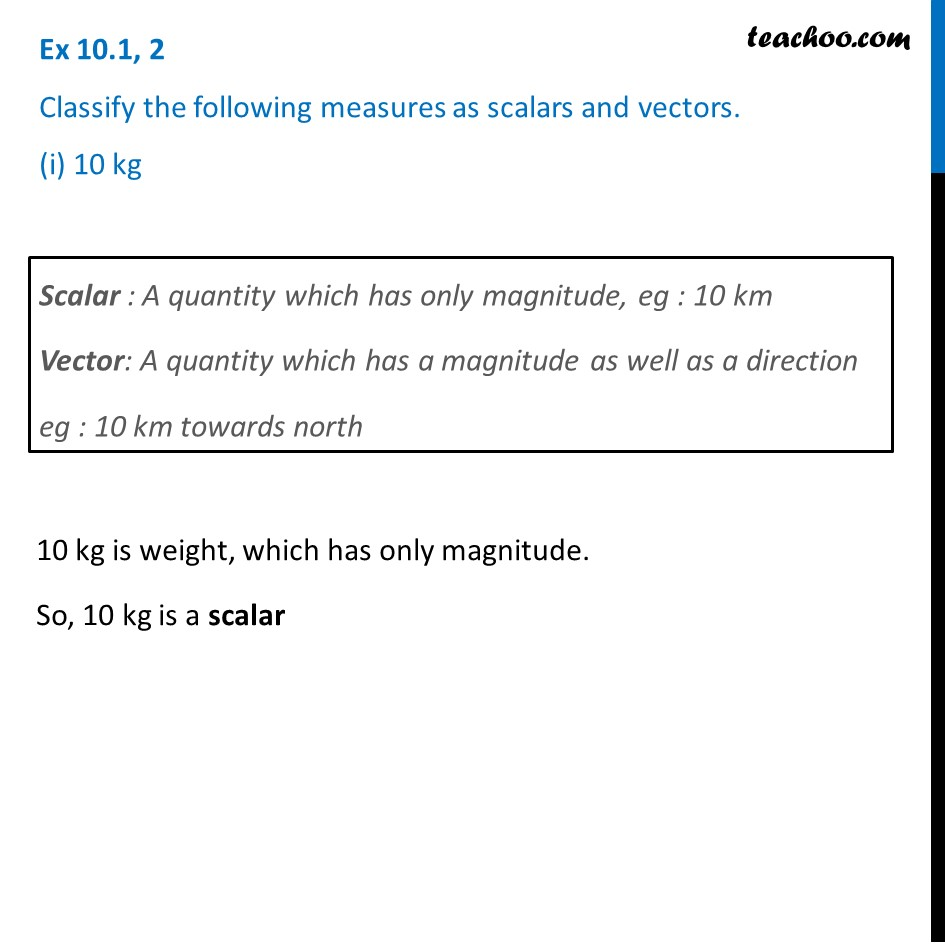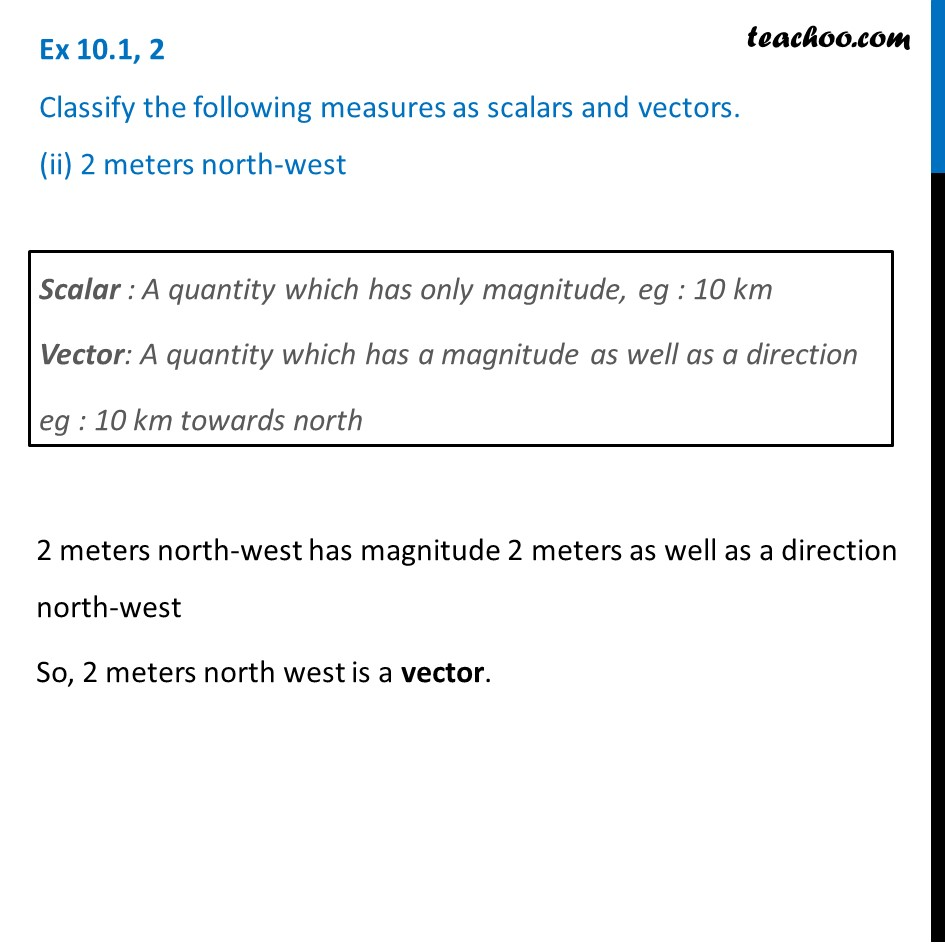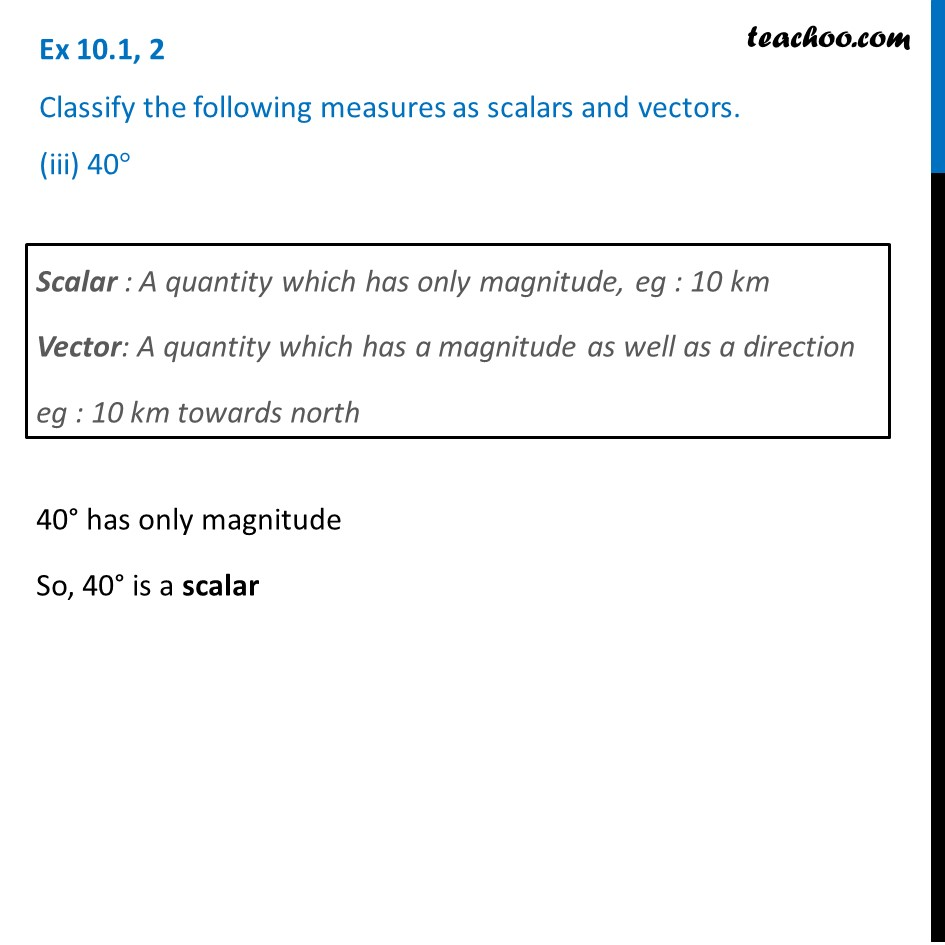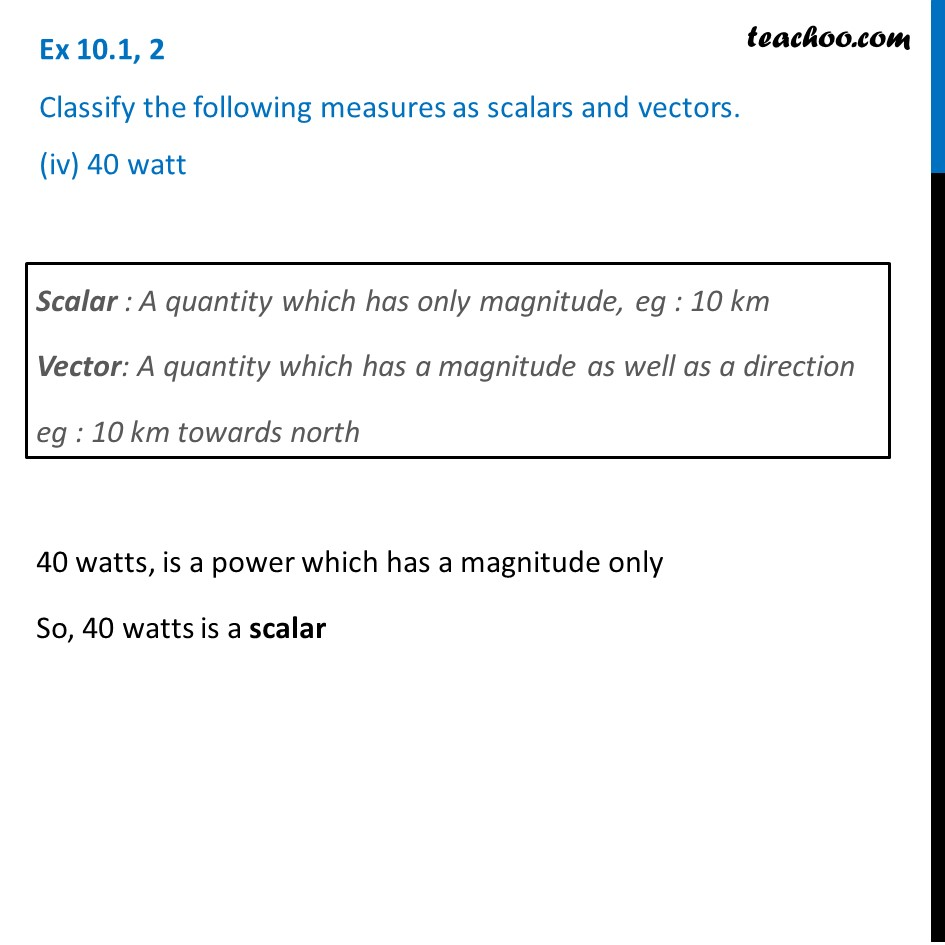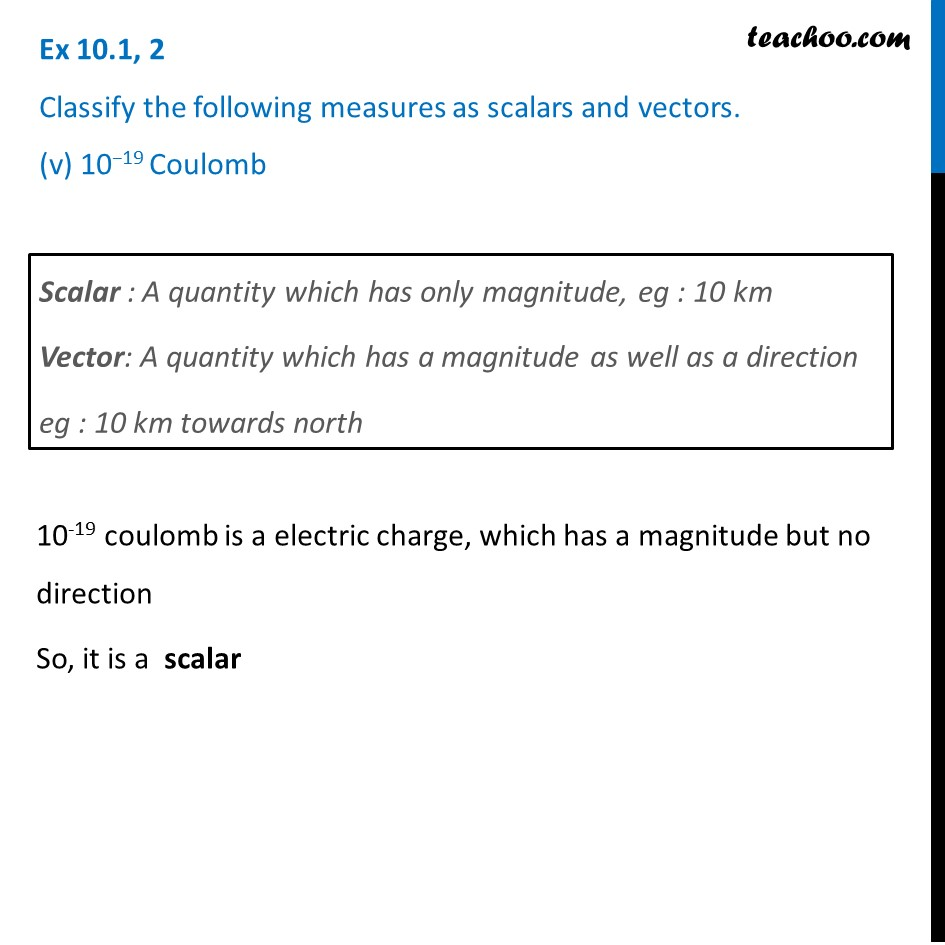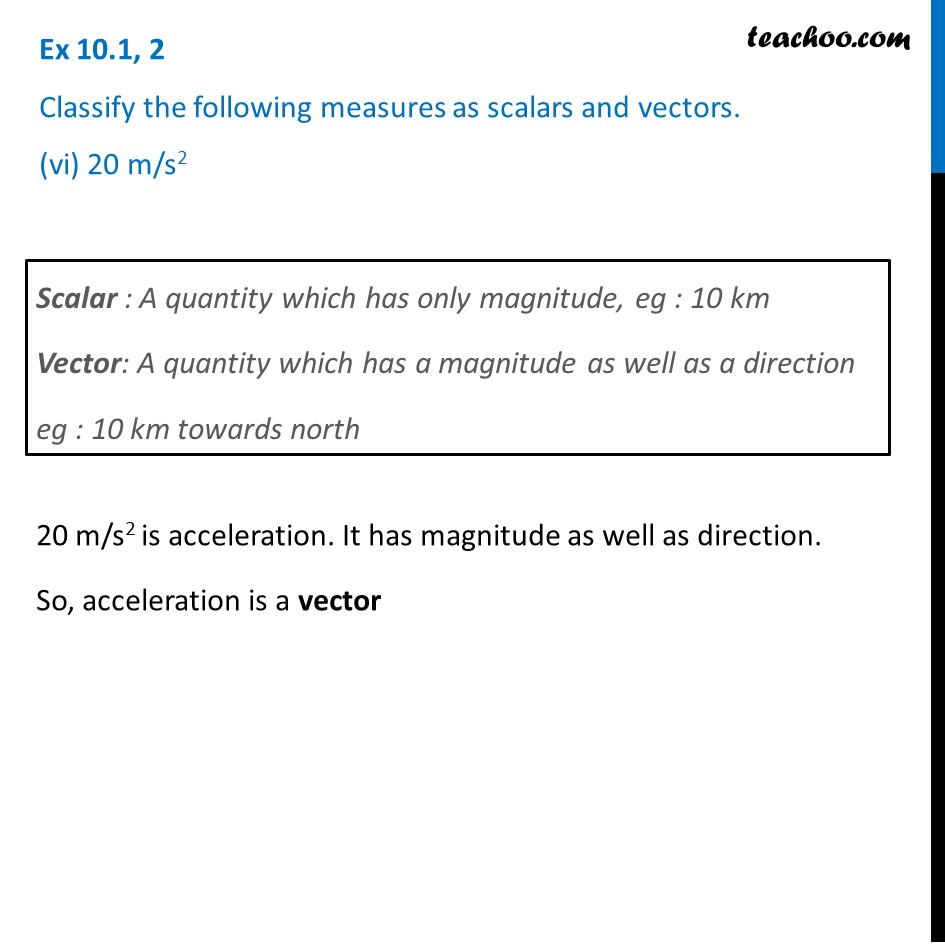Learn in your speed, with individual attention - Teachoo Maths 1-on-1 Class

### Transcript

Ex 10.1, 2 Classify the following measures as scalars and vectors. (i) 10 kgScalar : A quantity which has only magnitude, eg : 10 km Vector: A quantity which has a magnitude as well as a direction eg : 10 km towards north 10 kg is weight, which has only magnitude. So, 10 kg is a scalar Ex 10.1, 2 Classify the following measures as scalars and vectors. (ii) 2 meters north-westScalar : A quantity which has only magnitude, eg : 10 km Vector: A quantity which has a magnitude as well as a direction eg : 10 km towards north 2 meters north-west has magnitude 2 meters as well as a direction north-west So, 2 meters north west is a vector. Ex 10.1, 2 Classify the following measures as scalars and vectors. (iii) 40° Scalar : A quantity which has only magnitude, eg : 10 km Vector: A quantity which has a magnitude as well as a direction eg : 10 km towards north 40° has only magnitude So, 40° is a scalar Ex 10.1, 2 Classify the following measures as scalars and vectors. (iv) 40 wattScalar : A quantity which has only magnitude, eg : 10 km Vector: A quantity which has a magnitude as well as a direction eg : 10 km towards north 40 watts, is a power which has a magnitude only So, 40 watts is a scalar Ex 10.1, 2 Classify the following measures as scalars and vectors. (v) 10−19 CoulombScalar : A quantity which has only magnitude, eg : 10 km Vector: A quantity which has a magnitude as well as a direction eg : 10 km towards north 10-19 coulomb is a electric charge, which has a magnitude but no direction So, it is a scalar Ex 10.1, 2 Classify the following measures as scalars and vectors. (vi) 20 m/s2Scalar : A quantity which has only magnitude, eg : 10 km Vector: A quantity which has a magnitude as well as a direction eg : 10 km towards north 20 m/s2 is acceleration. It has magnitude as well as direction. So, acceleration is a vector